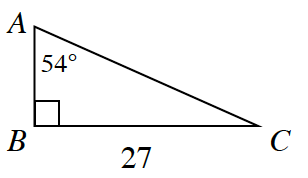### Home > CCG > Chapter 8 > Lesson 8.1.5 > Problem8-66

8-66.

Multiple Choice: Approximate the length of $\overline{AB}$.

1. $15.87$

1. $21.84$

1. $37.16$

1. $19.62$

1. none of theseUse the tangent ratio, explained in Lesson 4.1.4.
Tangent of an angle is equal to the opposite divided by the adjacent.